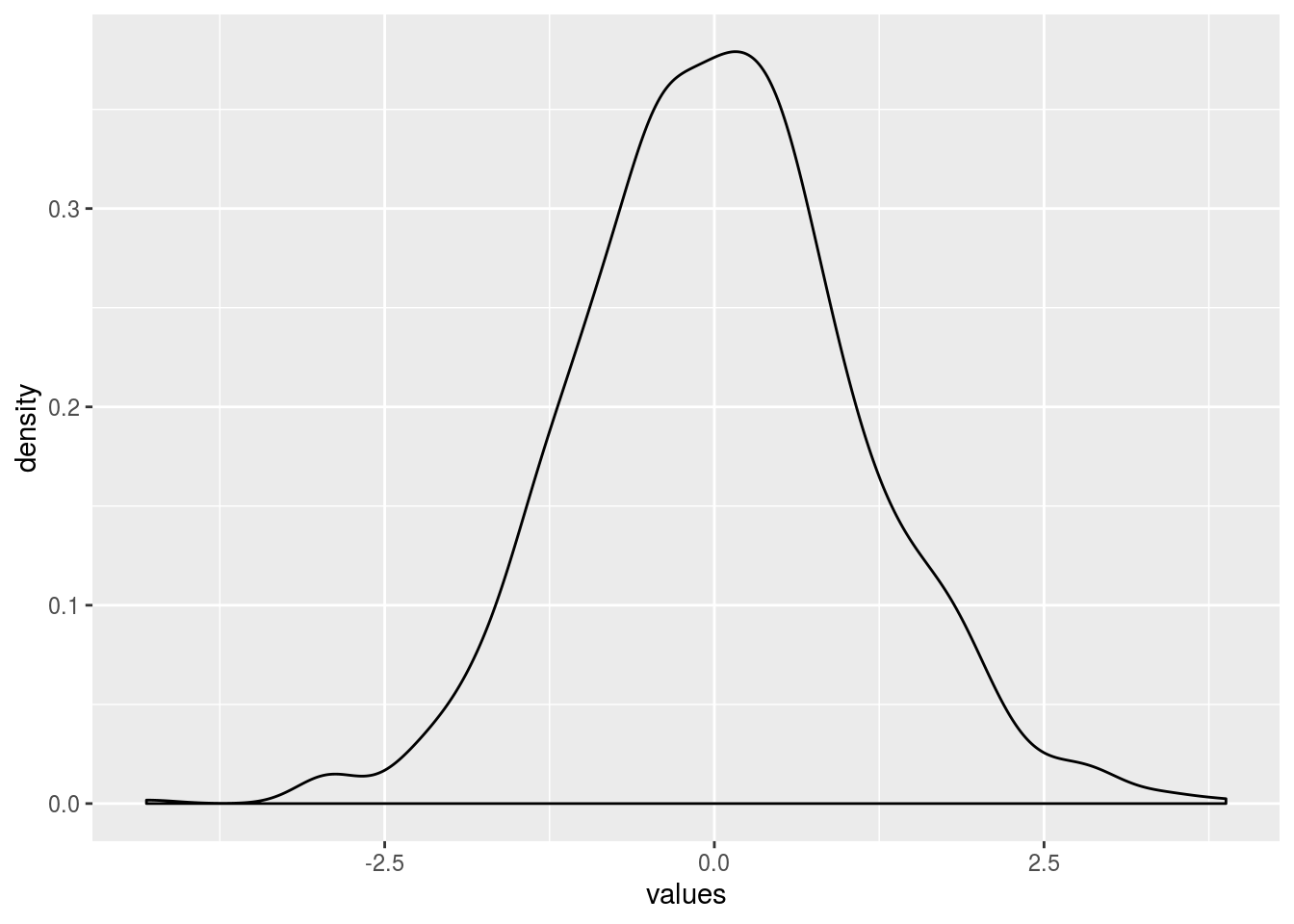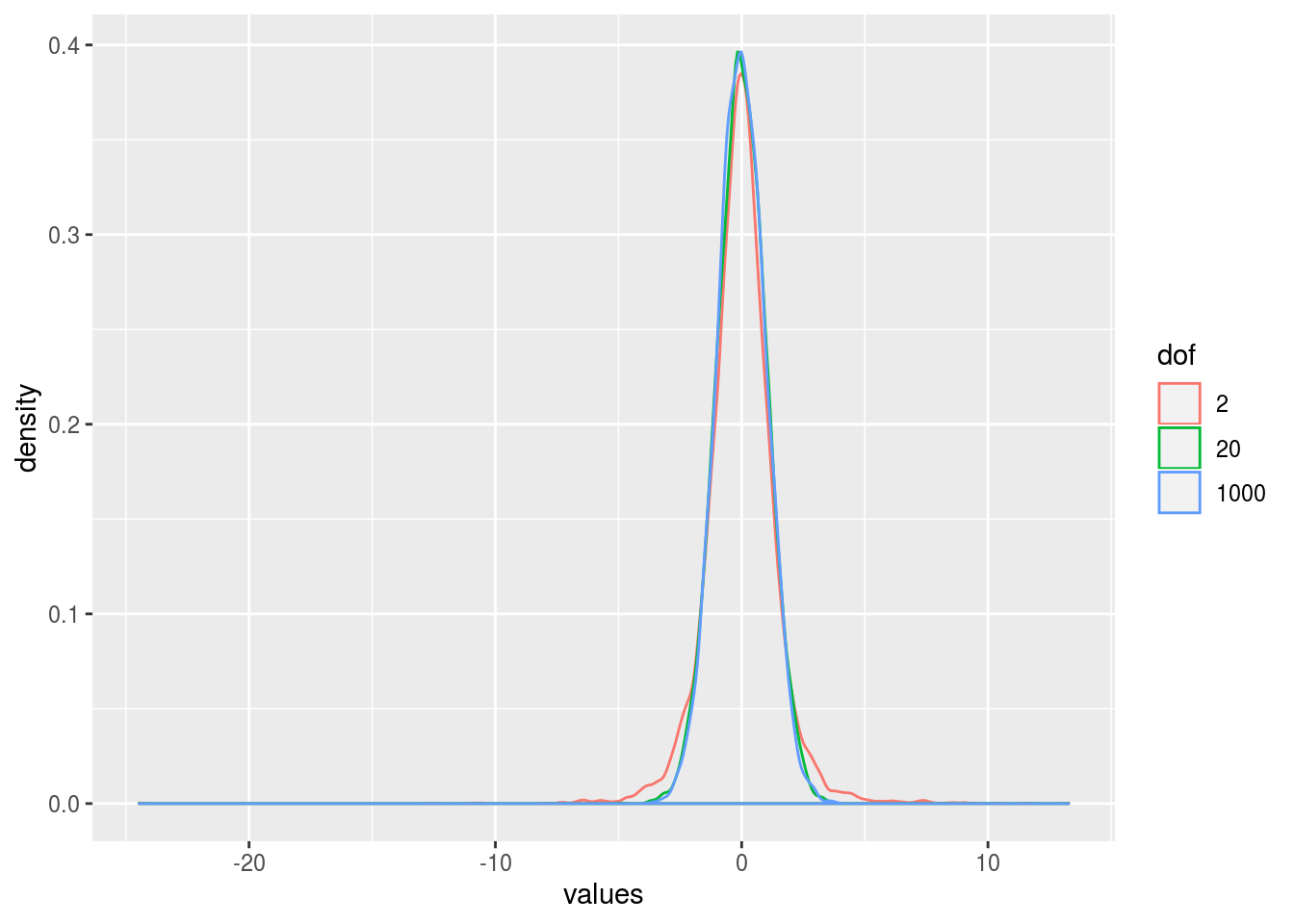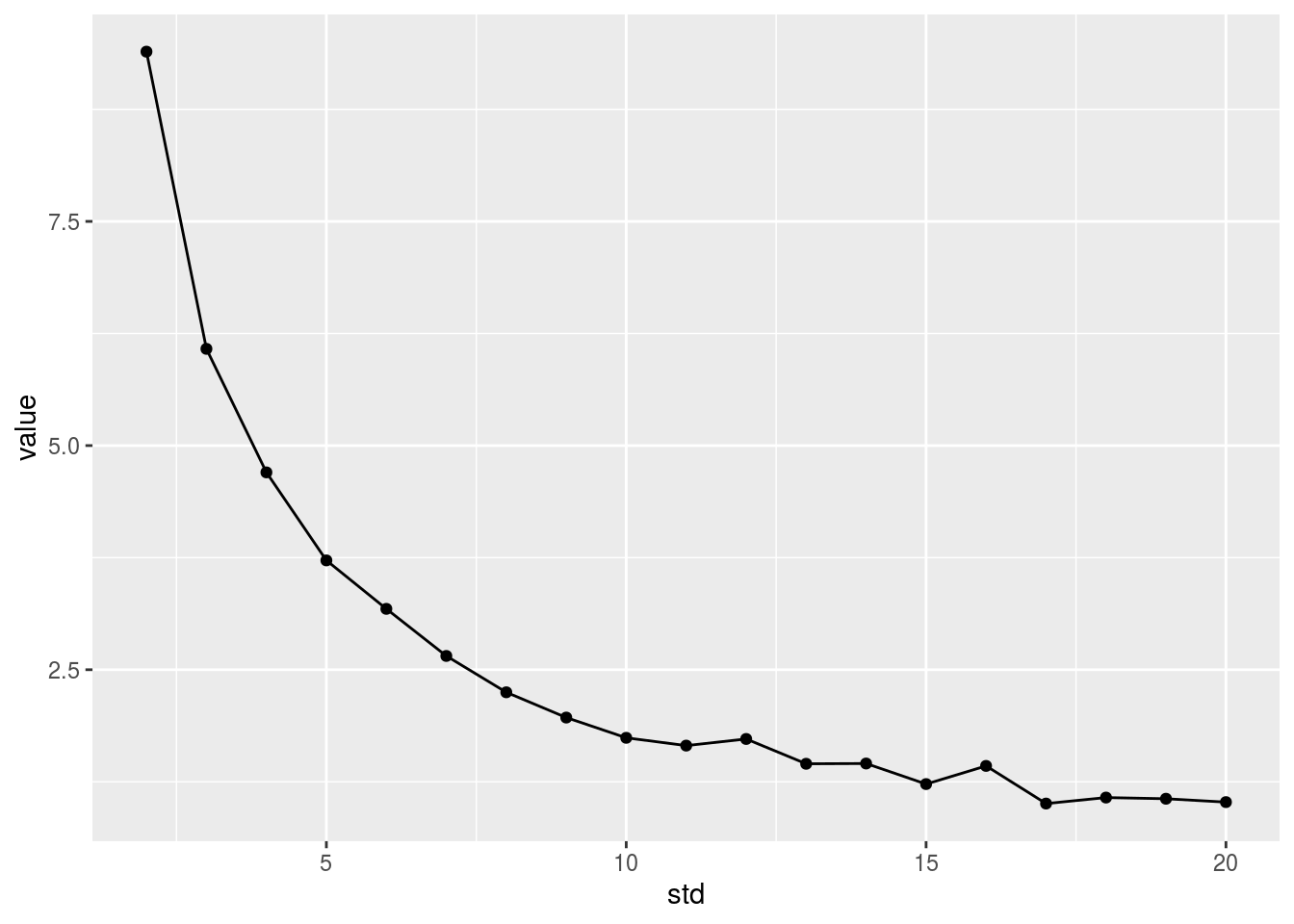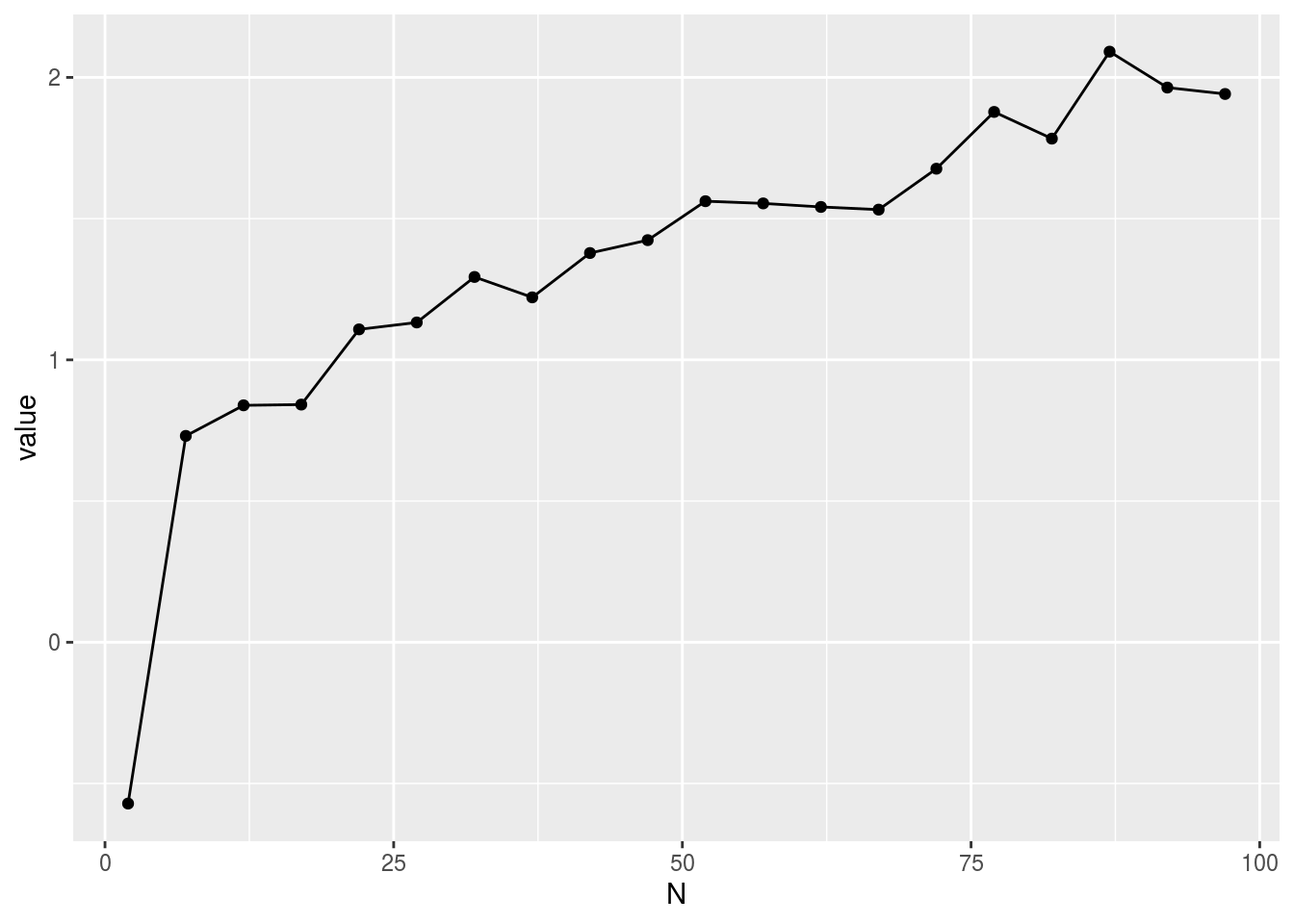In this lab we are going to dive deeper into one of the most common inferential tests used in statistics: the t-test.

Generally, there are 3 kinds of t-tests: the one-sample t-test, the paired sample t-test and the independent sample t-test. Each of those tests has its own uses but they all share a common idea: divide a measure of an effect by a measure of an error. The bigger the effect, the bigger the statistic, and the bigger the error, the smaller the statistic.

# One sample t-test

Let’s begin with a very simple scenario: You developed a drug that can improve memory (again) and you want to test this drug. So you take a very validated memory test and use it. You know that the population mean in this test is 70, but the standard deviation is unknown. You decided to test this drug on a sample of 20 students in BC, and you got a sample mean of 74 in this test and a sample standard deviation of 10. What can you conclude?

Let’s first simulate those scores and then we’ll conduct the test:

population_mean <- 70
sample_size <- 20
sample_mean <- 74
sample_std <- 10
sample_scores <- rnorm(n=sample_size, mean=sample_mean, sd=sample_std)

Now given this particular set of simulated scores, let’s see how we can perform a single sample t-test

t.test(sample_scores, mu = population_mean)
##
##  One Sample t-test
##
## data:  sample_scores
## t = 2.5599, df = 19, p-value = 0.01915
## alternative hypothesis: true mean is not equal to 70
## 95 percent confidence interval:
##  70.84746 78.44524
## sample estimates:
## mean of x
##  74.64635

According to the result I see here at this simulation, the t-value is 0.31 and the p-value = 0.76 (your results may differ) – so we clearly faild to reject the null hypothesis.

The actual calculation of a t-test is as follwos: divide the mean difference between the sample mean and the population mean (our measure of the effect) by the standard error of the null distribution (our measure of the error). Formally:

measure_of_effect <- mean(sample_scores) - population_mean
measure_of_error  <- sd(sample_scores) / sqrt(sample_size)
t_obt <- measure_of_effect / measure_of_error
t_obt
##  2.559936

Which gives us the same t-value we observed before.

# Independent samples t-test

The second type of t-test is the independent samples t-test. Here, you have two samples that are independent (i.e., not the same group) and you want to test if the difference between those two samples is significant or not. This particular test is actually the same test we used in the power analysis.

Let’s simulate some data and see how it works:

sample_size <- 20
sample_1_mean <- 70
sample_1_std <- 20
sample_2_mean <- 75
sample_2_std <- 20
sample_1_scores <- rnorm(n=sample_size, mean=sample_1_mean, sd=sample_1_std)
sample_2_scores <- rnorm(n=sample_size, mean=sample_2_mean, sd=sample_2_std)

Now let’s see how we can run a t-test:

t.test(sample_1_scores, sample_2_scores, var.equal = TRUE)
##
##  Two Sample t-test
##
## data:  sample_1_scores and sample_2_scores
## t = -1.0346, df = 38, p-value = 0.3074
## alternative hypothesis: true difference in means is not equal to 0
## 95 percent confidence interval:
##  -21.952024   7.103229
## sample estimates:
## mean of x mean of y
##  66.15069  73.57508

Which gives us a very similar output to what we had before.

The actual calculation of this test is again about dividing a measure of effect by a measure of error. The measure of the effect is the difference between the two means, and the measure of error is the average standard error of the two samples. Let’s see how that works:

measure_of_effect <- mean(sample_1_scores) - mean(sample_2_scores)
pooled_variance <- (sum((sample_1_scores-mean(sample_1_scores))^2) +
sum((sample_2_scores-mean(sample_2_scores))^2)) / (sample_size*2 -2)
measure_of_error  <- sqrt((pooled_variance/sample_size)+(pooled_variance/sample_size))
t_obt <- measure_of_effect / measure_of_error
t_obt
##  -1.034574

# Paired t-test

Finally, lets look at paired t-test. In this case, we have two dependent measures from the same sample and we want to decide if they are different. The difference in the code is only one more input to the t-test call. Let’s use the same code we used before:

sample_size <- 20
sample_1_mean <- 70
sample_1_std <- 20
sample_2_mean <- 75
sample_2_std <- 20
sample_1_scores <- rnorm(n=sample_size, mean=sample_1_mean, sd=sample_1_std)
sample_2_scores <- rnorm(n=sample_size, mean=sample_2_mean, sd=sample_2_std)

And now we can use the paired t-test:

t.test(sample_1_scores, sample_2_scores, paired = TRUE, var.equal = TRUE)
##
##  Paired t-test
##
## data:  sample_1_scores and sample_2_scores
## t = -1.3981, df = 19, p-value = 0.1782
## alternative hypothesis: true difference in means is not equal to 0
## 95 percent confidence interval:
##  -22.598193   4.498619
## sample estimates:
## mean of the differences
##               -9.049787

Note that in all of those analysis, we can assign the t-test call into a variable and query different sub-variables such as the statistic, the p-value etc. For example:

result <- t.test(sample_1_scores, sample_2_scores, paired = TRUE, var.equal = TRUE)
result$statistic # t-value ## t ## -1.398055 result$p.value   # p-value
##  0.1782052
result$parameter # df  ## df ## 19 # The t-distribution In the z-test, we have been dealing with the normal distribution and we had good times because we always know or assume the population standard deviation. When we no longer have a population standard deviation, we need to use the t-test and hence we now deal with the t-distribution. But with R, it is not as bad as it sounds. We can simply make a null distribution from random numbers and then come up with any value we need (just as we did with the z-test and normal distribution). Let’s generate a distribution of t-values and see how it looks like with ggplot2: ts <- c() sample_size <- 10 for (i in 1:1000){ ts[i] <- t.test(rnorm(n=sample_size, mean=0, sd=1), rnorm(n=sample_size, mean=0, sd=1))$statistic
}
library(ggplot2)
ggplot(data.frame(values=ts), aes(x=values)) + geom_density()And how we are ready to answer any probability question with t-test to calculate the critical values and obtain any probability. What is the probability that a t-value is above or below 2.5?

mean(ts > 2.5 | ts < -2.5)
##  0.028

Actually, this will be the distirubiton we use to calculate the confidence intervals as well.

Another issue is that this probability distribution is actually conditioned on the sample size (or more accurately, the degrees of freedom). Each degree of freedom makes a distinct t-distribution. As the degrees of freedom approaches infinity, the t-distribution approximate the normal distribution.

Let’s see if those claims are true

dfs <- c(2, 20, 1000)
ts <- c()
n_sims <- 10000
for (d in 1:length(dfs)){
df<-dfs[d]
sample_size <- df + 1
for (i in 1:n_sims){
ts <- c(ts, t.test(rnorm(n=sample_size), rnorm(n=sample_size))$statistic) } } ts_dof_df <- data.frame(values = ts, dof = as.factor(rep(dfs, each=n_sims))) ggplot(ts_dof_df, aes(x=values, color=dof)) + geom_density()# Effect of some parameters on the t-value Let’s take the single sample t-test. What is the effect of the standard deviation on the obtained t-value? Let’s make a simulation to see what happens as we change the standard deviation of the distribution we sample from: ts_vs_std <- c() population_mean <- 70 sample_size <- 20 sample_mean <- 74 n_exps <- 100 sample_std_vector <- c(2:20) for (ind in 1:length(sample_std_vector)){ sample_std <- sample_std_vector[ind] ts <- c() for (t_ind in 1:n_exps){ sample_scores <- rnorm(n=sample_size, mean=sample_mean, sd=sample_std) ts[t_ind] <- t.test(sample_scores, mu = population_mean)$statistic
}
ts_vs_std[ind] <- mean(ts)
}

Now let’s make a quick visual to see what is going on:

library(ggplot2)
ggplot(data.frame(value=ts_vs_std, std=sample_std_vector), aes(x=std, y=value)) +
geom_point()+ geom_line()Let’s see how the sample size affect the t-value

ts_vs_N <- c()
population_mean <- 70
sample_std <- 20
sample_mean <- 74
n_exps <- 100
sample_size_vector <- seq(2,100,5)
for (ind in 1:length(sample_size_vector)){
sample_size <- sample_size_vector[ind]
ts <- c()
for (t_ind in 1:n_exps){
sample_scores <- rnorm(n=sample_size, mean=sample_mean, sd=sample_std)
ts[t_ind] <- t.test(sample_scores, mu = population_mean)\$statistic
}
ts_vs_N[ind] <- mean(ts)
}

Now let’s make a quick visual to see what is going on:

library(ggplot2)
ggplot(data.frame(value=ts_vs_N, N=sample_size_vector), aes(x=N, y=value)) +
geom_point()+ geom_line()# Exercises

Use simulations to determine the effect of the following on the t-value (from an independent sample t-test): The sample size, the standard deviations of the estimates, the effect size. Use the same sample size and standard deviation for both groups. Which of those seem to increase the t-value?